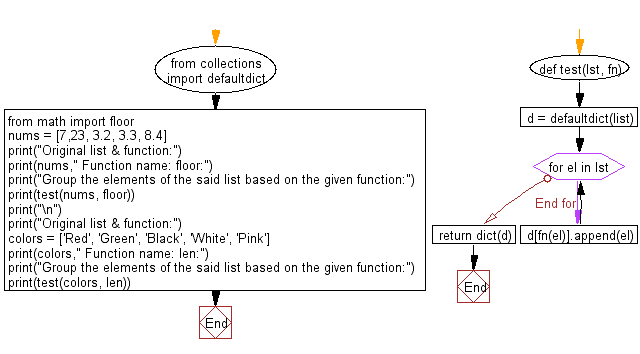﻿ Python: Groups the elements of a given list based on the given function - w3resource# Python: Groups the elements of a given list based on the given function

## Python dictionary: Exercise-69 with Solution

Write a Python program to group the elements of a given list based on the given function.

• Use collections.defaultdict to initialize a dictionary.
• Use fn in combination with a for loop and dict.append() to populate the dictionary.
• Use dict() to convert it to a regular dictionary.

Sample Solution:

Python Code:

``````from collections import defaultdict
from math import floor
def test(lst, fn):
d = defaultdict(list)
for el in lst:
d[fn(el)].append(el)
return dict(d)
nums = [7,23, 3.2, 3.3, 8.4]
print("Original list & function:")
print(nums," Function name: floor:")
print("Group the elements of the said list based on the given function:")
print(test(nums, floor))
print("\n")
print("Original list & function:")
colors = ['Red', 'Green', 'Black', 'White', 'Pink']
print(colors," Function name: len:")
print("Group the elements of the said list based on the given function:")
print(test(colors, len))
```
```

Sample Output:

```Original list & function:
[7, 23, 3.2, 3.3, 8.4]  Function name: floor:
Group the elements of the said list based on the given function:
{7: , 23: , 3: [3.2, 3.3], 8: [8.4]}

Original list & function:
['Red', 'Green', 'Black', 'White', 'Pink']  Function name: len:
Group the elements of the said list based on the given function:
{3: ['Red'], 5: ['Green', 'Black', 'White'], 4: ['Pink']}
```

Flowchart:## Visualize Python code execution:

The following tool visualize what the computer is doing step-by-step as it executes the said program:

Python Code Editor:

Have another way to solve this solution? Contribute your code (and comments) through Disqus.

What is the difficulty level of this exercise?

Test your Programming skills with w3resource's quiz.

﻿

## Python: Tips of the Day

Decapitalizes the first letter of a string:

Example:

```def tips_decapitalize(s, upper_rest=False):
return s[:1].lower() + (s[1:].upper() if upper_rest else s[1:])
print(tips_decapitalize('PythonTips'))
print(tips_decapitalize('PythonTips', True))
```

Output:

```pythonTips
pYTHONTIPS
```